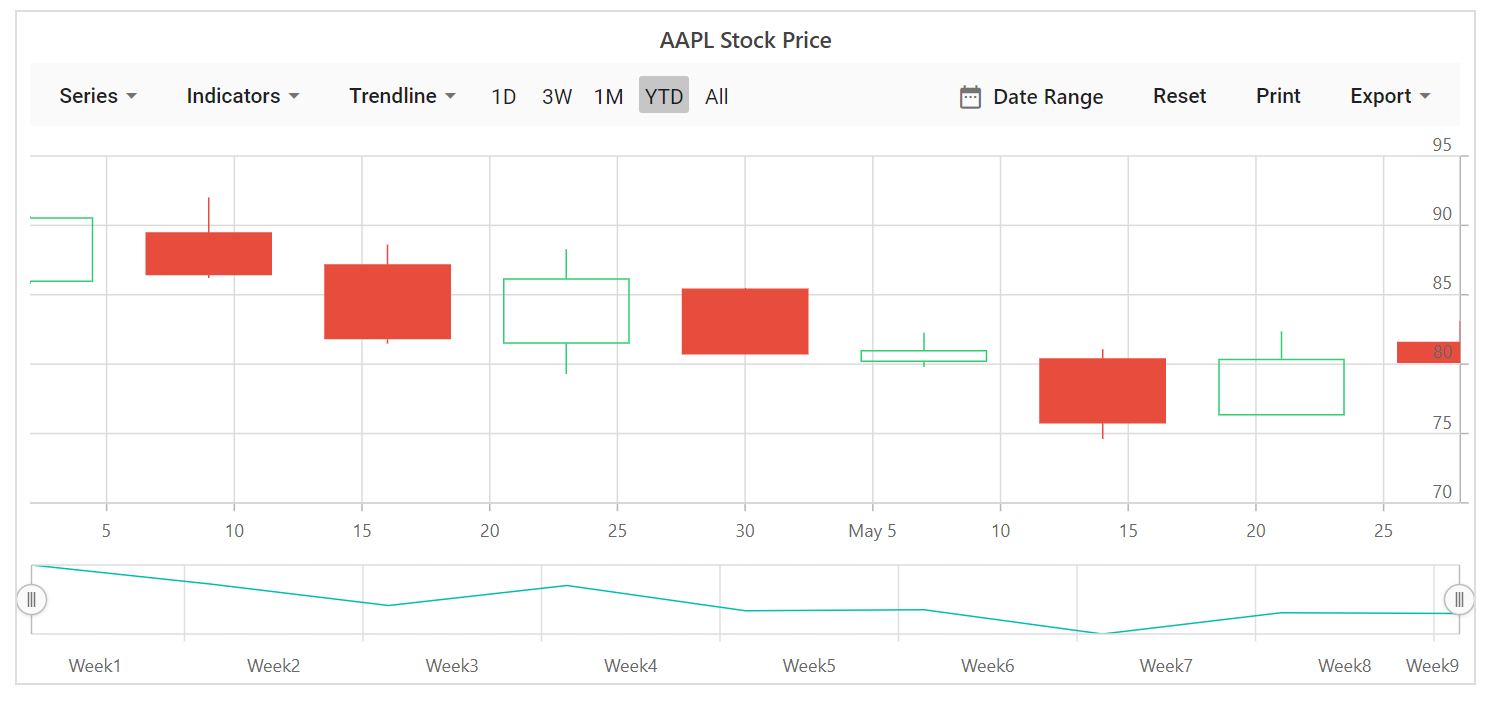Search results

# Series Types in Blazor Stock Chart component

28 Jul 2021 / 1 minute to read

Stock Chart supports 6 major types of series namely `Line`, `Spline`, `Hilo`, `HiloOpenClose`, `Hollow Candle` and `Candle`. By using the series dropdown button you can navigate between the above listed series types.

Line

To render a line series, use series `Type` as `Line`.

line.razor
``````@using Syncfusion.Blazor.Charts

<SfStockChart Title="AAPL Stock Price">
<StockChartSeriesCollection>
<StockChartSeries DataSource="@StockDetails" Type="ChartSeriesType.Line" XName="Date" YName="Y">
</StockChartSeries>
</StockChartSeriesCollection>
</SfStockChart>

@code {

public class ChartData
{
public DateTime Date;
public Double Y;
}
public List<ChartData> StockDetails = new List<ChartData>
{
new ChartData { Date = new DateTime(2012, 04, 02), Y= 100},
new ChartData { Date = new DateTime(2012, 04, 09), Y= 10},
new ChartData { Date = new DateTime(2012, 04, 16), Y= 500},
new ChartData { Date = new DateTime(2012, 04, 23), Y= 80},
new ChartData { Date = new DateTime(2012, 04, 30), Y= 200},
new ChartData { Date = new DateTime(2012, 05, 07), Y= 600},
new ChartData { Date = new DateTime(2012, 05, 14), Y= 50},
new ChartData { Date = new DateTime(2012, 05, 21), Y= 700},
new ChartData { Date = new DateTime(2012, 05, 28), Y= 90}
};
}``````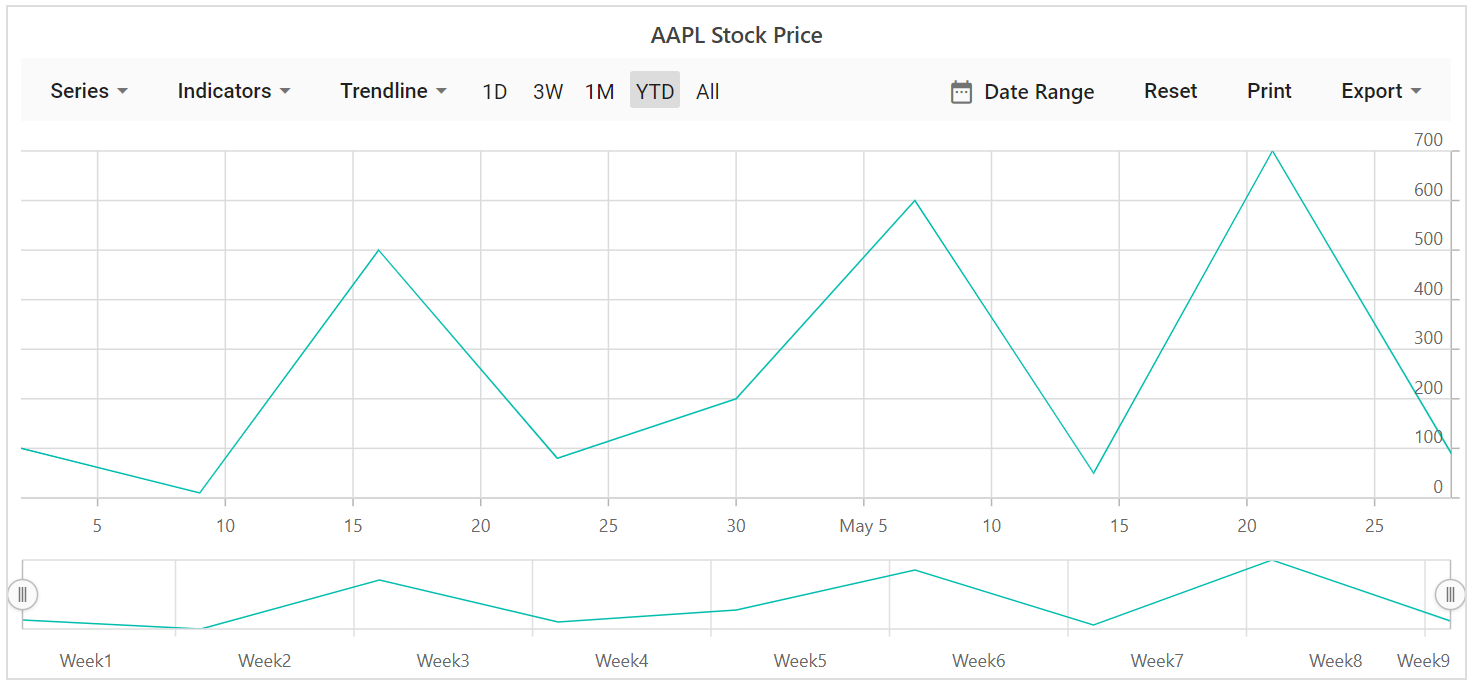Spline

To render a spline series, use series `Type` as `Spline`.

spline.razor
``````@using Syncfusion.Blazor.Charts

<SfStockChart Title="AAPL Stock Price">
<StockChartSeriesCollection>
<StockChartSeries DataSource="@StockDetails" Type="ChartSeriesType.Spline" XName="Date" YName="Y">
</StockChartSeries>
</StockChartSeriesCollection>
</SfStockChart>

@code {

public class ChartData
{
public DateTime Date;
public Double Y;
}
public List<ChartData> StockDetails = new List<ChartData>
{
new ChartData { Date = new DateTime(2012, 04, 02), Y= 100},
new ChartData { Date = new DateTime(2012, 04, 09), Y= 10},
new ChartData { Date = new DateTime(2012, 04, 16), Y= 500},
new ChartData { Date = new DateTime(2012, 04, 23), Y= 80},
new ChartData { Date = new DateTime(2012, 04, 30), Y= 200},
new ChartData { Date = new DateTime(2012, 05, 07), Y= 600},
new ChartData { Date = new DateTime(2012, 05, 14), Y= 50},
new ChartData { Date = new DateTime(2012, 05, 21), Y= 700},
new ChartData { Date = new DateTime(2012, 05, 28), Y= 90}
};
}``````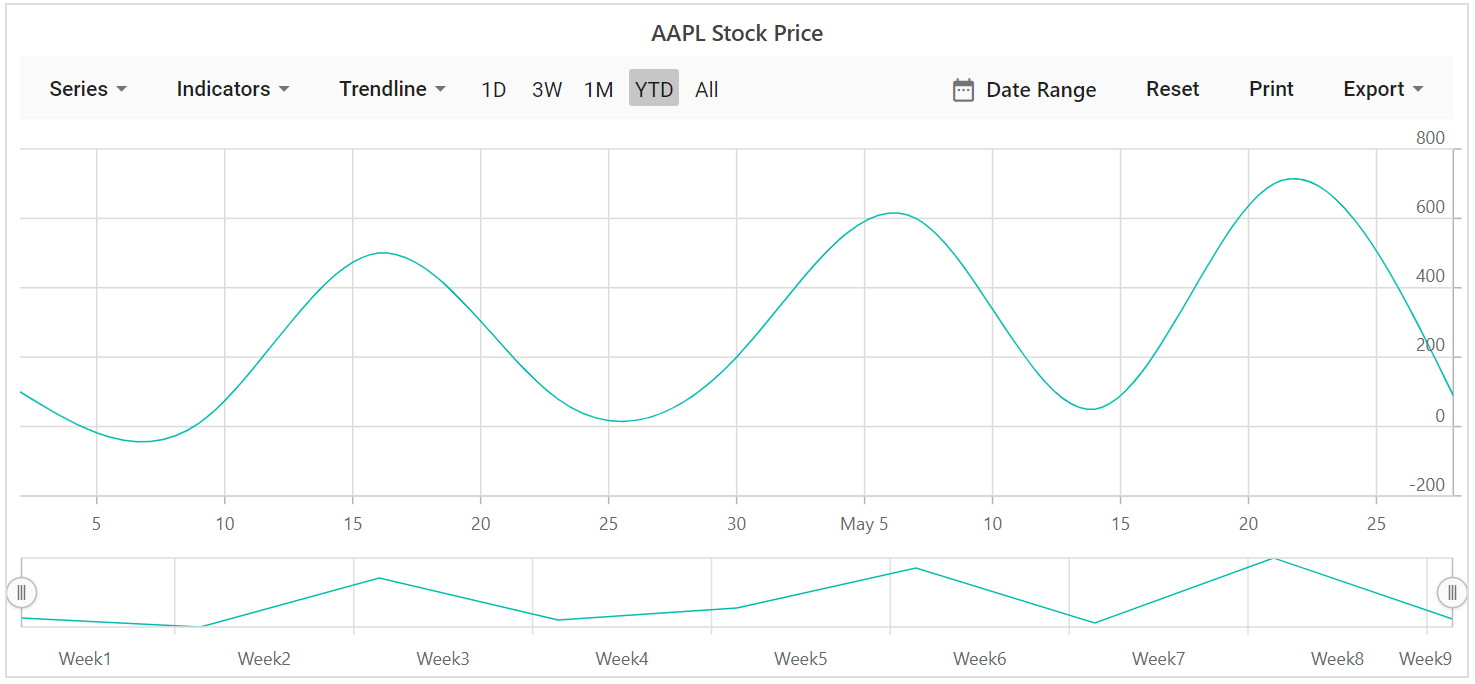HollowCandle

To render a hollowcandle series, use series `Type` as `Candle` and set `EnableSolidCandle` as false.

Hilo

To render a hilo series, use series `Type` as `Hilo`.

hilo.razor
``````@using Syncfusion.Blazor.Charts

<SfStockChart Title="AAPL Stock Price">
<StockChartSeriesCollection>
<StockChartSeries DataSource="@StockDetails" Type="ChartSeriesType.Hilo" XName="Date" High="High" Low="Low">
</StockChartSeries>
</StockChartSeriesCollection>
</SfStockChart>

@code {

public class StockChartData
{
public DateTime Date;
public Double Open;
public Double Low;
public Double Close;
public Double High;
public Double Volume;
}
public List<StockChartData> StockDetails = new List<StockChartData>
{
new StockChartData { Date = new DateTime(2012, 04, 02), Open = 85.9757, High = 90.6657, Low = 85.7685, Close = 90.5257, Volume = 660187068},
new StockChartData { Date = new DateTime(2012, 04, 09), Open = 89.4471, High = 92, Low = 86.2157, Close = 86.4614, Volume = 912634864},
new StockChartData { Date = new DateTime(2012, 04, 16), Open = 87.1514, High = 88.6071, Low = 81.4885, Close = 81.8543, Volume = 1221746066},
new StockChartData { Date = new DateTime(2012, 04, 23), Open = 81.5157, High = 88.2857, Low = 79.2857, Close = 86.1428, Volume = 965935749},
new StockChartData { Date = new DateTime(2012, 04, 30), Open = 85.4, High =  85.4857, Low = 80.7385, Close = 80.75, Volume = 615249365},
new StockChartData { Date = new DateTime(2012, 05, 07), Open = 80.2143, High = 82.2685, Low = 79.8185, Close = 80.9585, Volume = 541742692},
new StockChartData { Date = new DateTime(2012, 05, 14), Open = 80.3671, High = 81.0728, Low = 74.5971, Close = 75.7685, Volume = 708126233},
new StockChartData { Date = new DateTime(2012, 05, 21), Open = 76.3571, High = 82.3571, Low = 76.2928, Close = 80.3271, Volume = 682076215},
new StockChartData { Date = new DateTime(2012, 05, 28), Open = 81.5571, High = 83.0714, Low = 80.0743, Close = 80.1414, Volume = 480059584},
};
}``````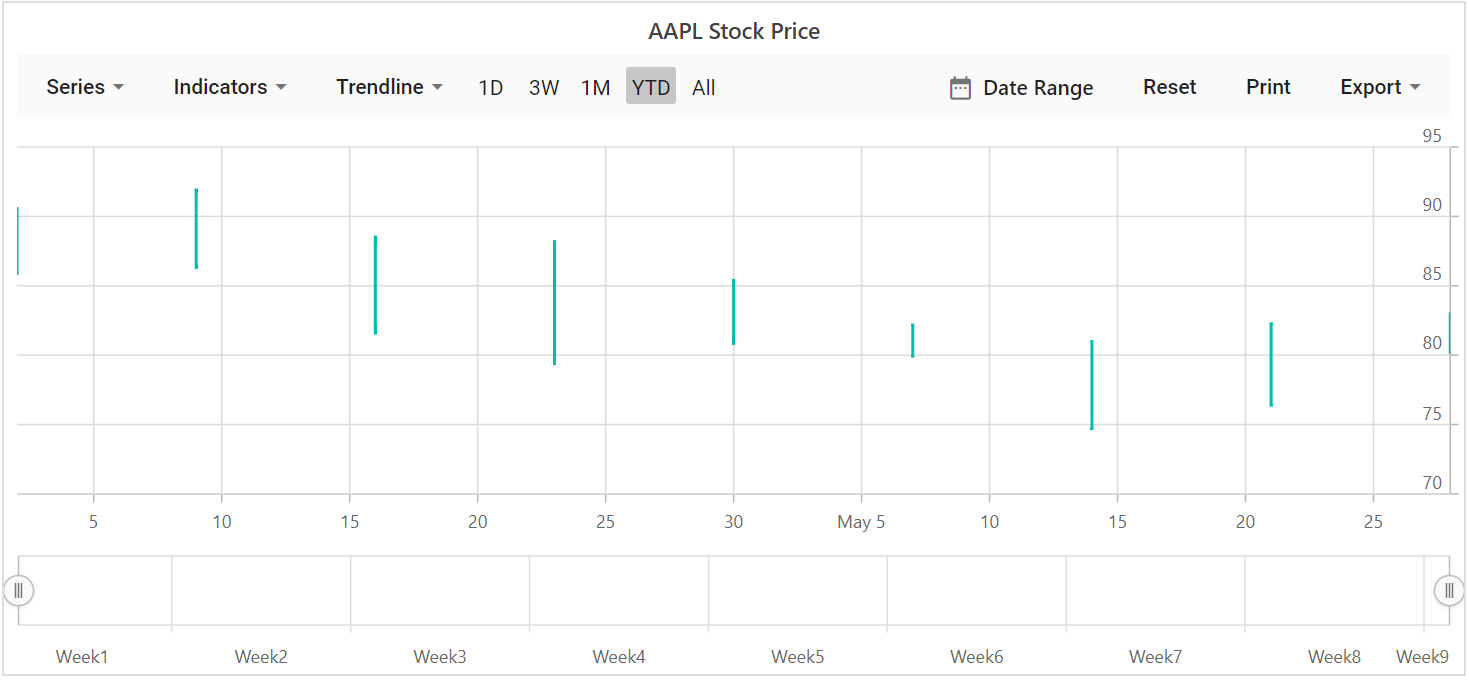HiloOpenClose

To render a HiloOpenClose series, use series `Type` as `HiloOpenClose`.

hiloopenclose.razor
``````@using Syncfusion.Blazor.Charts

<SfStockChart Title="AAPL Stock Price">
<StockChartSeriesCollection>
<StockChartSeries DataSource="@StockDetails" Type="ChartSeriesType.HiloOpenClose" XName="Date" High="High" Low="Low">
</StockChartSeries>
</StockChartSeriesCollection>
</SfStockChart>

@code {

public class StockChartData
{
public DateTime Date;
public Double Open;
public Double Low;
public Double Close;
public Double High;
public Double Volume;
}
public List<StockChartData> StockDetails = new List<StockChartData>
{
new StockChartData { Date = new DateTime(2012, 04, 02), Open = 85.9757, High = 90.6657, Low = 85.7685, Close = 90.5257, Volume = 660187068},
new StockChartData { Date = new DateTime(2012, 04, 09), Open = 89.4471, High = 92, Low = 86.2157, Close = 86.4614, Volume = 912634864},
new StockChartData { Date = new DateTime(2012, 04, 16), Open = 87.1514, High = 88.6071, Low = 81.4885, Close = 81.8543, Volume = 1221746066},
new StockChartData { Date = new DateTime(2012, 04, 23), Open = 81.5157, High = 88.2857, Low = 79.2857, Close = 86.1428, Volume = 965935749},
new StockChartData { Date = new DateTime(2012, 04, 30), Open = 85.4, High =  85.4857, Low = 80.7385, Close = 80.75, Volume = 615249365},
new StockChartData { Date = new DateTime(2012, 05, 07), Open = 80.2143, High = 82.2685, Low = 79.8185, Close = 80.9585, Volume = 541742692},
new StockChartData { Date = new DateTime(2012, 05, 14), Open = 80.3671, High = 81.0728, Low = 74.5971, Close = 75.7685, Volume = 708126233},
new StockChartData { Date = new DateTime(2012, 05, 21), Open = 76.3571, High = 82.3571, Low = 76.2928, Close = 80.3271, Volume = 682076215},
new StockChartData { Date = new DateTime(2012, 05, 28), Open = 81.5571, High = 83.0714, Low = 80.0743, Close = 80.1414, Volume = 480059584},
};
}``````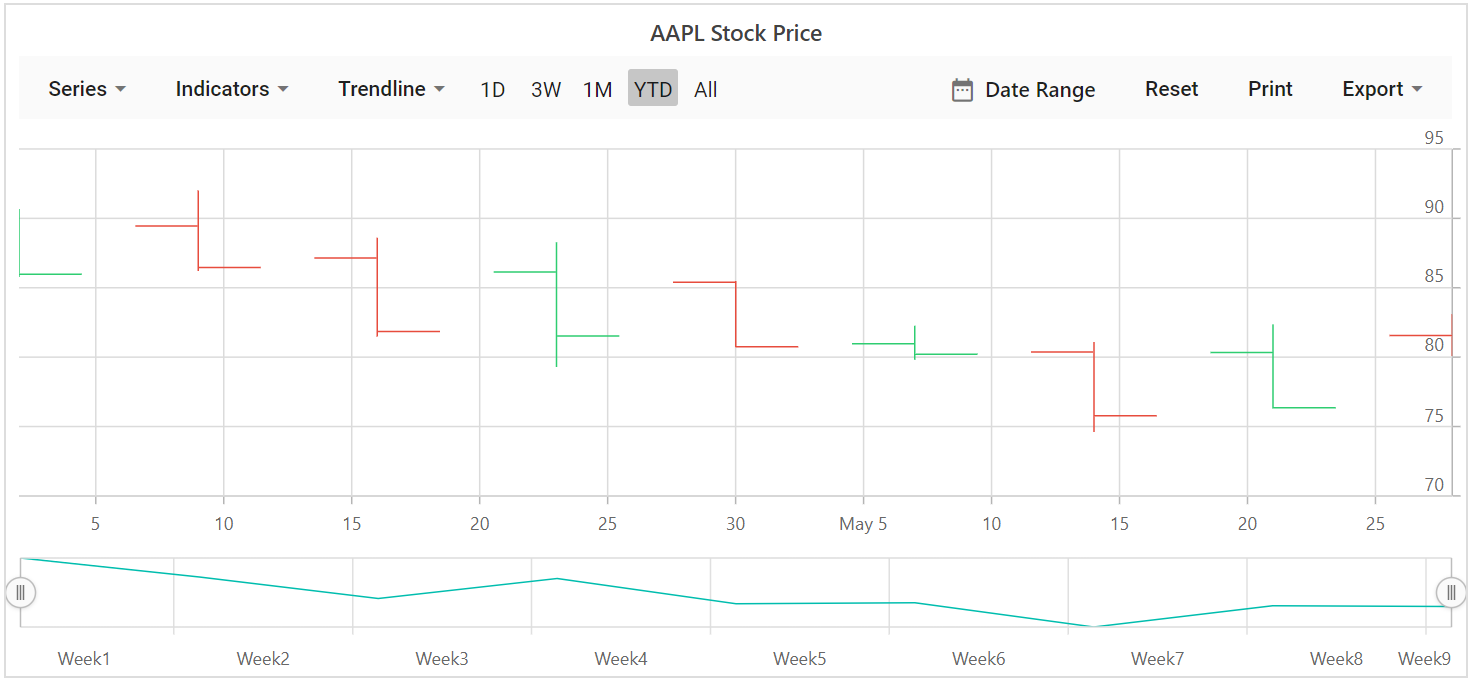Candle

To render a candle series, use series `Type` as `Candle`.

candle.razor
``````@using Syncfusion.Blazor.Charts

<SfStockChart Title="AAPL Stock Price">
<StockChartSeriesCollection>
<StockChartSeries DataSource="@StockDetails" Type="ChartSeriesType.Candle" XName="Date" High="High" Low="Low" Open="Open" Close="Close" Volume="Volume" Name="Google"></StockChartSeries>
</StockChartSeriesCollection>
</SfStockChart>
@code {

public class ChartData
{
public DateTime Date;
public Double Y;
}
public List<ChartData> StockDetails = new List<ChartData>
{
new ChartData { Date = new DateTime(2012, 04, 02), Y= 100},
new ChartData { Date = new DateTime(2012, 04, 09), Y= 10},
new ChartData { Date = new DateTime(2012, 04, 16), Y= 500},
new ChartData { Date = new DateTime(2012, 04, 23), Y= 80},
new ChartData { Date = new DateTime(2012, 04, 30), Y= 200},
new ChartData { Date = new DateTime(2012, 05, 07), Y= 600},
new ChartData { Date = new DateTime(2012, 05, 14), Y= 50},
new ChartData { Date = new DateTime(2012, 05, 21), Y= 700},
new ChartData { Date = new DateTime(2012, 05, 28), Y= 90}
};
}``````